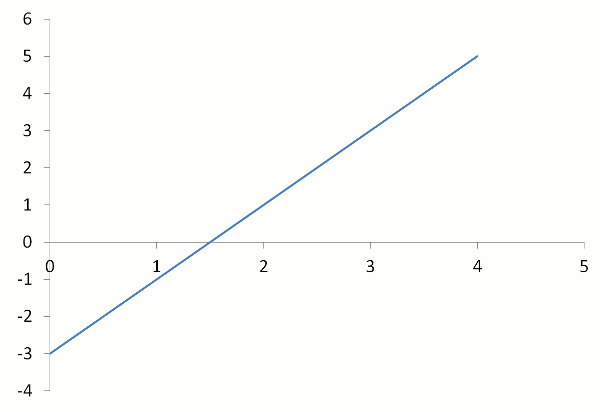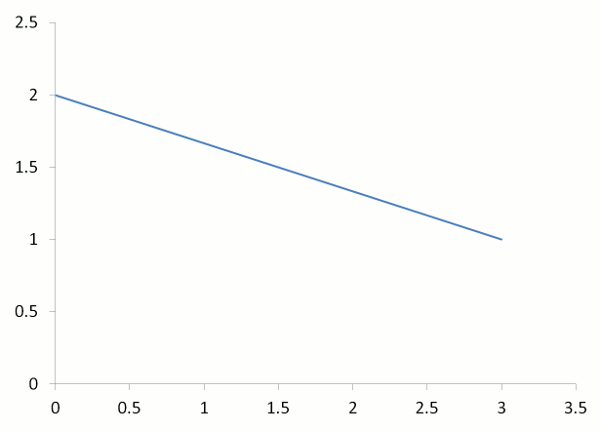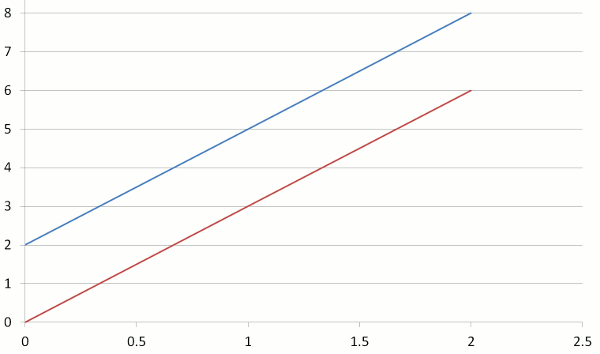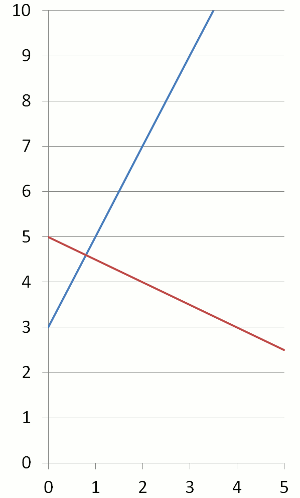where
Rise is the vertical height
Run is the horizontal height.

Civil engineers use this formula to work out the gradient of roads. Carpenters use this formula to calculate the slope of a step.The world's most dangerous road is the Yungas Road in Bolivia in South America. More than 200 people are killed each year. Not only is it steep in parts but it is also in an area of high blinding rainfall. Calculate the gradient (steepness) of a part of the road with a rise of 215 metres and a run of 500 metres.

 Gradient = Rise = 215 = 0.43 Run 500

 Gradient = m = y2 – y1 x2 – x1

where (x1, y1) and (x2, y2) are two points on a linear or straight-line graph.

Notice that the Rise is the difference between the y-values and the Run is the difference between the x-values.

What is the gradient between the points (2,1) and (4,5)?(x1, y1) = (2,1)
(x2, y2) = (4,5)

 Gradient = y2 – y1 = 5 – 1 = 4 = 2 x2 – x1 4 – 2 2

What is the gradient between the points (0,2) and (3,1)?(x1, y1) = (0,2)
(x2, y2) = (3,1)

 Gradient = y2 – y1 = 1 – 2 = –1 = –1⁄3 x2 – x1 3 – 0 3

Notice that when the gradient is negative, the graph slopes backwards.

Questions - Find the Gradient between Two Points

Q1. (5,6) and (7,12)
Q2. (3,6) and (5,2)

A1. 3
A2. –2

Lines that are parallel have the same gradient, m1 = m2

Example Four - Parallel Lines

The graphs of y = 3x (red line) and y = 3x + 2 (blue line) are shown in the diagram. What do you notice about their gradients (slopes)?Perpendicular lines are at right angles to each other.

When the gradients of perpendicular lines are multiplied, the resulting product is –1.

m1 × m2 = –1

Example Five - Perpendicular Lines

The graphs of y = 2x + 3 (blue line) and y = –12 x + 5 (red line) are shown in the diagram. What do you notice about these graphs?Perpendicular (at right angles) to each other

Example Six - Perpendicular Lines

If the gradient of a line is 2, what is the gradient of a line that is at right angles to it?

12
(2 multiplied by –12 equals –1)

Questions - Parallel or Perpendicular Lines

If the gradient of a line is 4, find the gradient of a line that is:
Q1. parallel
Q2. perpendicular.